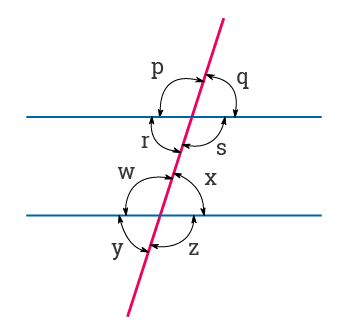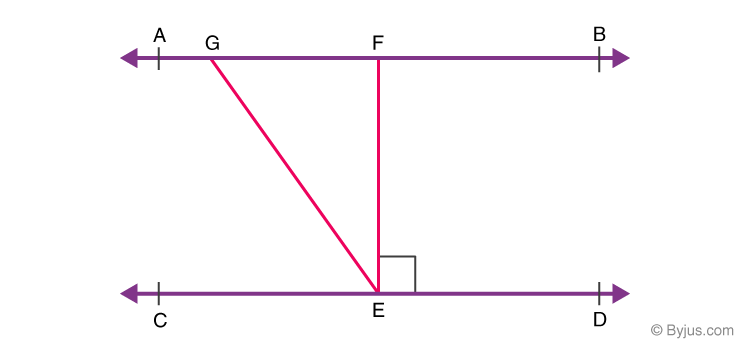# 9th Standard Math’s Chapter-6

9th Standard Math’s quiz Chapter-6 for solving the quiz students they will prepare exam, those who are  preparing yourself Competitive Exam like TET, JRF and NTA etc

1
Created on Bywefru

9th standard Math's Chapter 6

1 / 10

Q.51 A line joining two endpoints are called:

2 / 10

Q.52 An acute angle is:

3 / 10

Q.53 A reflex angle is:

4 / 10

Q.54 A straight angle is equal to:

5 / 10

Q.55 Two angles whose sum is equal to 180° are called:

6 / 10

Q.56 Intersecting lines cut each other at:

7 / 10

Q.57 Two parallel lines intersect at:

8 / 10

Q.58 If two lines intersect each other, then the vertically opposite angles are:

9 / 10

Q.59 In the figure below, which of the following are corresponding angle pairs:10 / 10

Q.60

If AB || CD, EF ⊥ CD and ∠GED = 135° as per the figure given below.